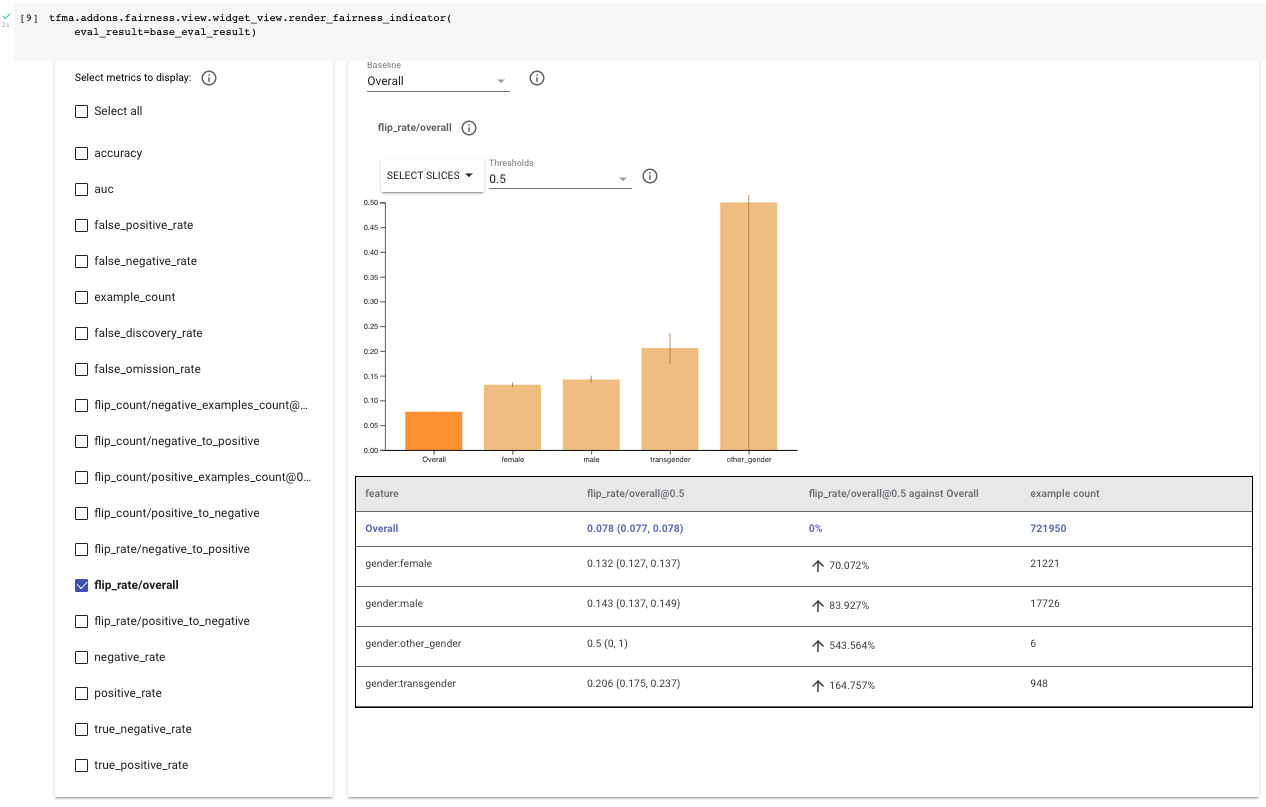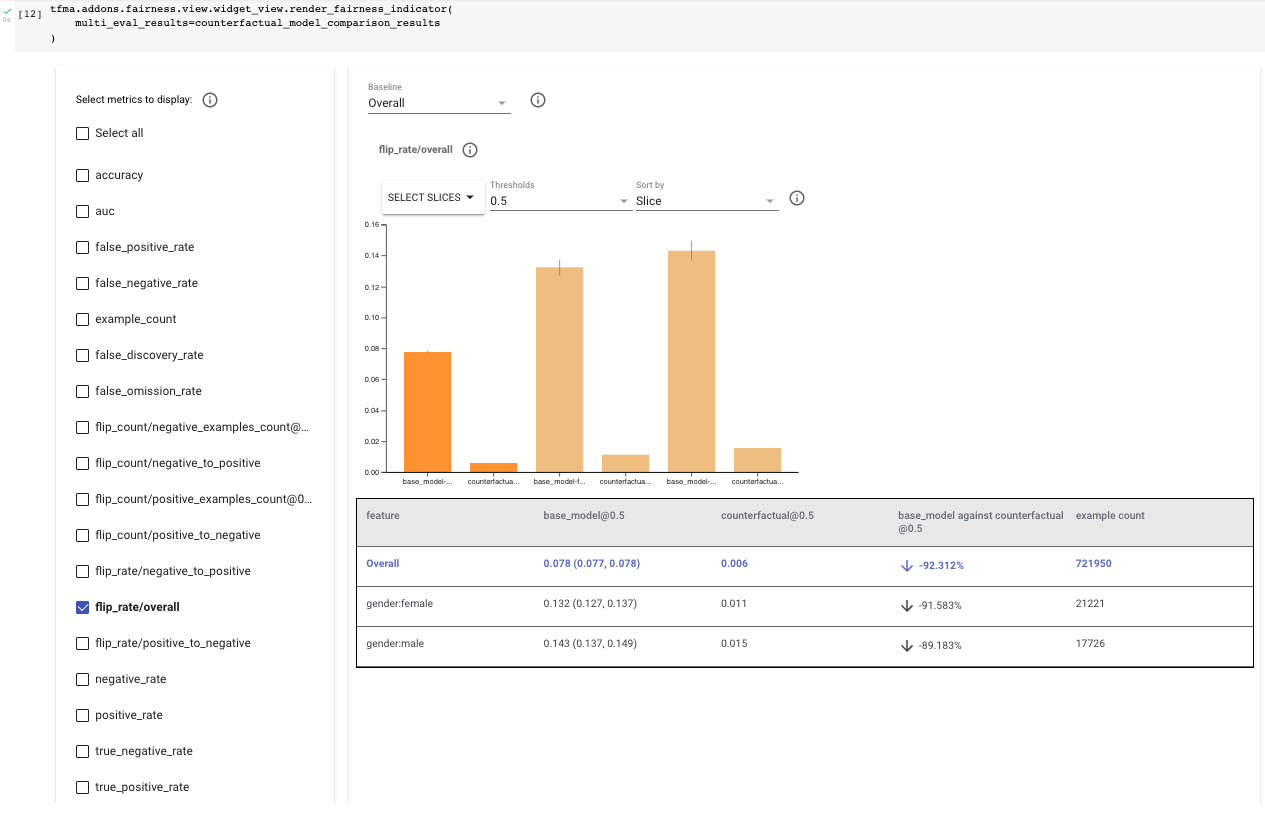# Using Counterfactual Logit Pairing with Keras

This notebook shows you how to train a text classifier to identify offensive content and use Counterfactual Logit Pairing (CLP) to avoid having identity terms unfairly skew what is classified as offensive. This type of model attempts to identify content that is rude, disrespectful or otherwise likely to make someone leave a discussion, and assigns the content a toxicity score. The CLP technique can be used to identify and mitigate correlations between identity terms and the toxicity score, and is available as part of the TensorFlow Model Remediation Library.

After the initial launch of the Perspective API, users discovered a positive correlation between identity terms containing information on race or sexual orientation and the predicted toxicity score. For example, the phrase "I am a lesbian" received a toxicity score of 0.51, while “I am a man” received a lower toxicity score of 0.2. In this case, the identity terms were not being used pejoratively, so there should not be such a significant difference in the score.

Within this Colab, you will explore how to use CLP to train train a text classifier with a similar bias as the Perspective API and how to remediate the bias. You'll progress in following these steps:

1. Build a baseline model to classify the toxicity of text.
2. Create an instance of `CounterfactualPackedInputs` with the `original_input` and `counterfactual_data` to evaluate the model’s performance on flip rate and flip count to determine if intervention is needed.
3. Train with the CLP technique to avoid unintended correlation between model output and sensitive identity terms.
4. Evaluate the new model’s performance on the flip rate and flip count.

This tutorial demonstrates a minimal usage of the CLP technique. When evaluating a model's performance with respect to Responsible AI principles, consider that there are many more tools available:

## Setup

You begin by installing Fairness Indicators and TensorFlow Model Remediation.

````pip install --upgrade tensorflow-model-remediation`
`pip install --upgrade fairness-indicators`
```

Import all necessary components, including CLP and Fairness Indicators for evaluation.

``````import os
import requests
import tempfile
import zipfile

import numpy as np
import pandas as pd
import tensorflow as tf
import tensorflow_hub as hub
import tensorflow_model_analysis as tfma

# Import Counterfactuals.
from tensorflow_model_remediation import counterfactual

import pkg_resources
import importlib
``````
```<module 'pkg_resources' from '/tmpfs/src/tf_docs_env/lib/python3.7/site-packages/pkg_resources/__init__.py'>
```

You use the provided utility function below called `download_and_process_civil_comments_data` to download the preprocessed data and prepare the labels to match the model’s output shape. The function also downloads the data as TFRecords to make later evaluation quicker.

Set up the `comment_text` feature as input and `toxicity` as the label.

``````TEXT_FEATURE = 'comment_text'
LABEL = 'toxicity'
BATCH_SIZE = 512
``````

### Utility Functions

```Downloading data from https://storage.googleapis.com/civil_comments_dataset/train_df_processed.csv
345699197/345699197 [==============================] - 2s 0us/step
229970098/229970098 [==============================] - 1s 0us/step
324941336/324941336 [==============================] - 2s 0us/step
```

## Define and train the baseline model

To reduce runtime, you can use a pretrained model that will be loaded by default. It is a simple Keras sequential model with an initial embedding and convolution layers, outputting a toxicity prediction. If you prefer, you can change this and train from scratch using the utility function defined above to create the model.

``````use_pretrained_model = True

if use_pretrained_model:
ZIPPATH = 'baseline_model.zip'
DIRPATH = '/tmp/baseline_model'
with requests.get(URL, allow_redirects=True) as r:
with open(ZIPPATH, 'wb') as z:
z.write(r.content)

with zipfile.ZipFile(ZIPPATH, 'r') as zip_ref:
zip_ref.extractall('/')
DIRPATH, custom_objects={'KerasLayer' : hub.KerasLayer})

else:
loss = tf.keras.losses.BinaryCrossentropy()

baseline_model = (
create_keras_sequential_model())
baseline_model.compile(optimizer=optimizer, loss=loss,
metrics=['accuracy'])

baseline_model.fit(x=data_train[TEXT_FEATURE],
y=labels_train, batch_size=BATCH_SIZE,
epochs=1)
``````

To evaluate the original model's performance using Fairness Indicators you will need to save the model.

``````base_dir = tempfile.mkdtemp(prefix='saved_models')
baseline_model_location = os.path.join(base_dir, 'model_export_baseline')
baseline_model.save(baseline_model_location, save_format='tf')
``````
```WARNING:absl:Found untraced functions such as _jit_compiled_convolution_op, _jit_compiled_convolution_op, _jit_compiled_convolution_op while saving (showing 3 of 3). These functions will not be directly callable after loading.
INFO:tensorflow:Assets written to: /tmpfs/tmp/saved_modelsqkj6w5ix/model_export_baseline/assets
INFO:tensorflow:Assets written to: /tmpfs/tmp/saved_modelsqkj6w5ix/model_export_baseline/assets
```

## Determine if intervention is needed

Use CLP to try to reduce the flip rate and count for gender related terms in the dataset.

### Preparing `CounterfactualPackedInputs`

To use CLP, you will first need to create an instance of `CounterfactualPackedInputs`, which includes the `original_input` and `counterfactual_data`.

`CounterfactualPackedInputs` looks like the following:

``````CounterfactualPackedInputs(
original_input=(x, y, sample_weight),
counterfactual_data=(original_x, counterfactual_x,
counterfactual_sample_weight)
)
``````

`original_input` should be the original dataset that is used to train your Keras model. `counterfactual_data` should be a `tf.data.Dataset` with the original `x` values, a corresponding counterfactual value, and the sample weight. The `counterfactual_x` value is nearly identical to the original value but with one or more of the sensitive attributes removed or replaced. This dataset is used to pair the loss function between the original value and the counterfactual value with the goal of assuring that the model’s prediction doesn’t change when the sensitive attribute is different.

Here’s an example of what a `counterfactual_data` would look like if you remove the term “gay”:

``````original_x: “I am a gay man”
counterfactual_x: “I am a man”
counterfactual_sample_weight”: 1
``````

If you are working with text, you can use the provided helper function `build_counterfactual_data` to create `counterfactual_data`. For all other data types, you need to provide `counterfactual_data` directly.

For an example of creating a `counterfactual_data` with `build_counterfactual_data`, see the Creating a Custom Counterfactual Dataset Colab.

In this example, you will remove a list of gender specific terms using `build_counterfactual_data`. You must only include non-pejorative terms, as pejorative terms should have a different toxicity score. Requiring equal predictions across examples with pejorative terms can accidentally harm the more vulnerable group.

``````sensitive_terms_to_remove = [
'aunt', 'boy', 'brother', 'dad', 'daughter', 'father', 'female', 'gay',
'girl', 'grandma', 'grandpa', 'grandson', 'grannie', 'granny', 'he',
'heir', 'her', 'him', 'his', 'hubbies', 'hubby', 'husband', 'king',
'mom', 'mother', 'mum', 'nephew', 'niece', 'prince', 'princess',
'queen', 'queens', 'she', 'sister', 'son', 'uncle', 'waiter',
'waitress', 'wife', 'wives', 'woman', 'women'
]

# Convert the Pandas DataFrame to a TF Dataset
dataset_train_main = tf.data.Dataset.from_tensor_slices(
(data_train[TEXT_FEATURE].values, labels_train))

counterfactual_data = counterfactual.keras.utils.build_counterfactual_data(
original_input=dataset_train_main,
sensitive_terms_to_remove=sensitive_terms_to_remove)

counterfactual_packed_input = counterfactual.keras.utils.pack_counterfactual_data(
dataset_train_main,
counterfactual_data).batch(BATCH_SIZE)
``````

## Calculate the Example Count, Flip Rate, and Flip Count

Next run Fairness Indicators to calculate the flip rate and flip count to see if the model is incorrectly associating some gender identity terms with toxicity. Running Fairness Indicators also enables you to calculate the example count to ensure that there are a sufficient number of examples to apply the technique. A flip is defined as a classifier giving a different prediction when the identity term in the example changes. Flip count measures the number of times the classifier gives a different decisio n if the identity term in a given example were changed. Flip rate measures the probability that the classifier gives a different decision if the identity term in a given example were changed.

``````def get_eval_results(model_location,
eval_result_path,
validate_tfrecord_file,
slice_selection='gender',
compute_confidence_intervals=True):
"""Get Fairness Indicators eval results."""
# Define slices that you want the evaluation to run on.
eval_config = text_format.Parse("""
model_specs {
label_key: '%s'
}
metrics_specs {
metrics {class_name: "AUC"}
metrics {class_name: "ExampleCount"}
metrics {class_name: "Accuracy"}
metrics {
class_name: "FairnessIndicators"
}
metrics {
class_name: "FlipRate"
config: '{ "counterfactual_prediction_key": "toxicity", '
'"example_id_key": 1 }'
}
}
slicing_specs {
feature_keys: '%s'
}
slicing_specs {}
options {
compute_confidence_intervals { value: %s }
disabled_outputs{values: "analysis"}
}
""" % (LABEL, slice_selection, compute_confidence_intervals),
tfma.EvalConfig())

eval_shared_model = tfma.default_eval_shared_model(
eval_saved_model_path=model_location, tags=[tf.saved_model.SERVING])

return tfma.run_model_analysis(
eval_shared_model=eval_shared_model,
data_location=validate_tfrecord_file,
eval_config=eval_config,
output_path=eval_result_path)

base_dir = tempfile.mkdtemp(prefix='eval')
eval_dir = os.path.join(base_dir, 'tfma_eval_result_no_cf')
base_eval_result = get_eval_results(
baseline_model_location,
eval_dir,
validate_tfrecord_file,
slice_selection='gender')
``````
```WARNING:absl:Tensorflow version (2.9.0-rc2) found. Note that TFMA support for TF 2.0 is currently in beta
WARNING:apache_beam.runners.interactive.interactive_environment:Dependencies required for Interactive Beam PCollection visualization are not available, please use: `pip install apache-beam[interactive]` to install necessary dependencies to enable all data visualization features.
WARNING:root:Make sure that locally built Python SDK docker image has Python 3.7 interpreter.
WARNING:apache_beam.io.tfrecordio:Couldn't find python-snappy so the implementation of _TFRecordUtil._masked_crc32c is not as fast as it could be.
WARNING:tensorflow:From /tmpfs/src/tf_docs_env/lib/python3.7/site-packages/tensorflow_model_analysis/writers/metrics_plots_and_validations_writer.py:109: tf_record_iterator (from tensorflow.python.lib.io.tf_record) is deprecated and will be removed in a future version.
Instructions for updating:
Use eager execution and:
`tf.data.TFRecordDataset(path)`
WARNING:tensorflow:From /tmpfs/src/tf_docs_env/lib/python3.7/site-packages/tensorflow_model_analysis/writers/metrics_plots_and_validations_writer.py:109: tf_record_iterator (from tensorflow.python.lib.io.tf_record) is deprecated and will be removed in a future version.
Instructions for updating:
Use eager execution and:
`tf.data.TFRecordDataset(path)`
```
``````# docs-infra: no-execute
eval_result=base_eval_result)
``````Examine the evaluation results, starting with the overall flip rate (“flip_rate/overall”). In this example, you consider four gender-related attributes within this dataset: “female”, “male”, “transgender”, and “other_gender”.

Start by checking the example count. “Other gender” and “transgender” have a low example count compare to the overall dataset; this is somewhat expected, as historically marginalized groups are often underrepresented in ML datasets. They also have wide confidence intervals, which indicates that the metrics calculated may not be representative. This notebook focuses on “female” and “male” subgroups because there is not sufficient data to apply the technique to “other gender” and “transgender”. It is important to evaluate the counterfactual fairness of the “other gender” and “transgender” groups. You can collect additional data to reduce the confidence intervals.

By selecting `flip_rate/overall` within Fairness Indicators, notice that the overall flip rate for females is about 13% and male is about 14%, which are both higher than the overall dataset of 8%. This means that the model is likely to change the classification based on the presence of the terms listed within `sensitive_terms_to_remove`.

You'll now CLP to try to reduce the flip rate and count for gender related terms in our dataset.

### Training and Evaluating the CLP Model

To train with CLP, pass in your original pretrained model, counterfactual loss, and data in the form of `CounterfactualPackedInputs`. Note that there are two optional parameters within `CounterfactualModel`, `loss_weight` and `loss` that you can adjust to tune your model.

Next compile the model normally (using the regular non-Counterfactual loss) and fit it to train.

``````counterfactual_weight = 1.0

base_dir = tempfile.mkdtemp(prefix='saved_models')
counterfactual_model_location = os.path.join(
base_dir, 'model_export_counterfactual')

counterfactual_model = counterfactual.keras.CounterfactualModel(
baseline_model,
loss=counterfactual.losses.PairwiseMSELoss(),
loss_weight=counterfactual_weight)

# Compile the model normally after wrapping the original model.
# Note that this means we use the baseline's model's loss here.
loss = tf.keras.losses.BinaryCrossentropy()
counterfactual_model.compile(optimizer=optimizer, loss=loss,
metrics=['accuracy'])

counterfactual_model.fit(counterfactual_packed_input,
epochs=1)

counterfactual_model.save_original_model(counterfactual_model_location,
save_format='tf')
``````
```2116/2116 [==============================] - 100s 46ms/step - total_loss: 0.2104 - counterfactual_loss: 5.2596e-04 - original_loss: 0.2099 - accuracy: 0.9273
WARNING:absl:Found untraced functions such as _jit_compiled_convolution_op, _jit_compiled_convolution_op, _jit_compiled_convolution_op while saving (showing 3 of 3). These functions will not be directly callable after loading.
INFO:tensorflow:Assets written to: /tmpfs/tmp/saved_modelsm5wp1q95/model_export_counterfactual/assets
INFO:tensorflow:Assets written to: /tmpfs/tmp/saved_modelsm5wp1q95/model_export_counterfactual/assets
```
``````# docs-infra: no-execute

def get_eval_results_counterfactual(
baseline_model_location,
counterfactual_model_location,
eval_result_path,
validate_tfrecord_file,
slice_selection='gender'):
"""Get Fairness Indicators eval results."""
eval_config = text_format.Parse("""
model_specs { name: 'original' label_key: '%s' }
model_specs { name: 'counterfactual' label_key: '%s' is_baseline: true }
metrics_specs {
metrics {class_name: "AUC"}
metrics {class_name: "ExampleCount"}
metrics {class_name: "Accuracy"}
metrics { class_name: "FairnessIndicators" }
metrics { class_name: "FlipRate" config: '{ "example_ids_count": 0 }' }
metrics { class_name: "FlipCount" config: '{ "example_ids_count": 0 }' }
}
slicing_specs { feature_keys: '%s' }
slicing_specs {}
options { disabled_outputs{ values: "analysis"} }
""" % (LABEL, LABEL, slice_selection,), tfma.EvalConfig())

eval_shared_models = [
tfma.default_eval_shared_model(
model_name='original',
eval_saved_model_path=baseline_model_location,
eval_config=eval_config,
tags=[tf.saved_model.SERVING]),
tfma.default_eval_shared_model(
model_name='counterfactual',
eval_saved_model_path=counterfactual_model_location,
eval_config=eval_config,
tags=[tf.saved_model.SERVING]),
]

return tfma.run_model_analysis(
eval_shared_model=eval_shared_models,
data_location=validate_tfrecord_file,
eval_config=eval_config,
output_path=eval_result_path)

counterfactual_eval_dir = os.path.join(base_dir, 'tfma_eval_result_cf')
counterfactual_eval_result = get_eval_results_counterfactual(
baseline_model_location,
counterfactual_model_location,
counterfactual_eval_dir,
validate_tfrecord_file)
``````

Evaluate the Counterfactual model by passing both the original and counterfactual model into Fairness Indicators together to get a side-by-side comparison. Once again, select “flip_rate/overall” within Fairness Indicators and compare the results for female and male between the two models. You should notice that the flip rate for overall, female, and male have all decreased by about 90%, which leaves the final flip rate for female at approximately 1.3% and male at approximately 1.4%.

Additionally, reviewing “flip_rate/negative_to_positive” and “flip_rate/positive_to_negative” you’ll notice that the model is still more likely to flip gender related content to toxic, but the total count has decreased by over 35%.

``````# docs-infra: no-execute
counterfactual_model_comparison_results = {
'base_model': base_eval_result,
'counterfactual': counterfactual_eval_result.get_results(),
}